# Python Itertools

## Python Programming Training Certification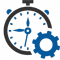Flexible Hours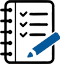100 Assignments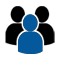Instructor Led online Training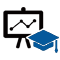50 LMS Access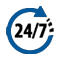24X7 Support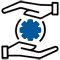100% Skill Level

### Enquire Now

4.9 out of 1000+ Ratings
Best Python Institute for Learning Python Course & Training, Live Project Training in Python with Django, Data Science and AI, Interview Assistance, Expert Coaching Trainers. Python Certification & Interview Assistance! Get free demo now!

### Course Overview

Python is one of the world’s top programming languages used today and Python training has become the most popular training across individuals. Training Basket’s Python Training & Certification course covers basic and advanced Python concepts and how to apply them in real-world applications.Python is a flexible and powerful open-source language that is easy to learn and consists of powerful libraries for data analysis and manipulation. Our Python training course content is curated by experts as per the standard Industry curriculum. The curriculum, coding challenges and real-life problems cover data operations in Python, strings, conditional statements, error handling, shell scripting, web scraping and the commonly used Python web framework Django. Take this Python training and certification course and become job-ready now.### Python Itertools

Itertool is one of the most amazing Python 3 standard libraries. This library has pretty much coolest functions and nothing wrong to say that it is the gem of the Python programing language. Python provides excellent documentation of the itertools but in this tutorial, we will discuss few important and useful functions or iterators of itertools.

The key thing about itertools is that the functions of this library are used to make memory-efficient and precise code.

Before learning the Python itertools, you should have knowledge of the Python iterator and generators. In this article, we will describe itertools for beginners are well as for professionals.

Introduction

According to the official definition of itertools, “this module implements a number of iterator building blocks inspired by constructs from APL, Haskell, and SML.” In simple words, the number of iterators can together create ‘iterator algebra’ which makes it possible to complete the complex task. The functions in itertools are used to produce more complex iterators. Let’s take an example: Python built-in zip() function accepts any number of arguments as iterable. It iterates over tuples and return their corresponding elements.

• a = [1,2,3]
b= [‘a’, ‘b’, ‘c’]
c = zip(a,b)
print(c)

Output:

• [(1, ‘a’), (2, ‘b’), (3, ‘c’)]

In the above code, we have passed two lists [1,2,3] and [‘a’, ‘b’, ‘c’] as iterable in zip() function. These lists return one element at a time. In Python, an element that implement .__iter__() or .__getitem__() method called iterable.

The Python iter() function is used to call on the iterable and return iterator object of the iterable.

• a = iter(‘Hello’)
print(a)

Output:

• <str_iterator object at 0x01505FA0>

The Python zip() function calls iter() on each of its argument and then calls next() by combining the result into tuple.

Note: If you are using the zip() function and map() function that means you are already using itertools. You don’t need to import it distinctly.

Types of Iterator

There are various types of iterator in itertools module. The list is given below:

• Infinite iterators
• Combinatoric iterators
• Terminating iterators

Infinite Iterators

In Python, any object that can implement for loop is called iterators. Lists, tuples, set, dictionaries, strings are the example of iterators but iterator can also be infinite and this type of iterator is called infinite iterator.

Iterator Argument Results
count(start,step) start, [step] start, start+step, step+2*step
cycle() P p0,p1,….plast
repeat() elem [,n] elem, elem, elem,….endlessly or upto n times
• count(start, stop): It prints from the start value to infinite. The step argument is optional, if the value is provided to the step then the number of steps will be skipped. Consider the following example:
• import itertools

for i in itertools.count(10,5):
if i == 50:
break
else:
print(i,end=” “)

Output:

• 10 15 20 25 30 35 40 45
• cycle(iterable): This iterator prints all value in sequence from the passed argument. It prints the values in a cyclic manner. Consider the following example:
• import itertools
temp = 0
for i in itertools.cycle(“123”):
if temp > 7:
break
else:
print(i,end=’ ‘)
temp = temp+1

Output:

• 1 2 3 1 2 3 1 2 3 1 2

Example – 2: Using next() function

• import itertools

val = [‘Java’, ‘T’, ‘Point’]

iter = itertools.cycle(val)

for i in range(6):
# Using next function
print(next(iter), end = ” “)

Output:

• Java T Point Java T Point
• repeat(val,num): As the name suggests, it repeatedly prints the passed value for infinite time. The num argument is optional. Consider the following example:
• import itertools
print(list(itertools.repeat(40,15)))

Output:

• [40, 40, 40, 40, 40, 40, 40, 40, 40, 40, 40, 40, 40, 40, 40]

Combinatoric iterators: The complex combinatorial constructs are simplified by the recursive generators. The permutations, combinations, and Cartesian products are the example of the combinatoric construct.

In Python, there are four types of combinatoric iterators:

• Product() – It is used to calculate the cartesian product of input iterable. In this function, we use the optional repeat keyword argument for computation of the product of an iterable with itself. The repeat keyword represents the number of repetitions. It returns output in the form of sorted tuples. Consider the following example:
• from itertools import product

print(“We are computing cartesian product using repeat Keyword Argument:”)
print(list(product([1, 2], repeat=2)))
print()

print(“We are computing cartesian product of the containers:”)
print(list(product([‘Java’, ‘T’, ‘point’], ‘5’)))
print()

print(“We are computing product of the containers:”)
print(list(product(‘CD’, [4, 5])))

Output:

• Computing cartesian product using repeat Keyword Argument:
[(1, 1), (1, 2), (2, 1), (2, 2)]

Computing cartesian product of the containers:
[(‘Java’, ‘5’), (‘T’, ‘5’), (‘point’, ‘5’)]

Computing product of the containers:
[(‘C’, 4), (‘C’, 5), (‘D’, 4), (‘D’, 5)]

• Permutations(): It is used to generate all possible permutation of an iterable. The uniqueness of each element depends upon their position instead of values. It accepts two argument iterable and group_size. If the value of group_size is none or not specified then group_size turns into length of the iterable.
• from itertools import permutations

print(“Computing all permutation of the following list”)
print(list(permutations([3,”Python”],2)))
print()

print(“Permutations of following string”)
print(list(permutations(‘AB’)))
print()

print(“Permutation of the given container is:”)
print(list(permutations(range(4),2)))

Output:

• Computing all permutation of the following list
[(3, ‘Python’), (‘Python’, 3)]

Permutations of following string
[(‘A’, ‘B’), (‘B’, ‘A’)]

Permutation of the given container is:
[(0, 1), (0, 2), (0, 3), (1, 0), (1, 2), (1, 3), (2, 0), (2, 1), (2, 3), (3, 0), (3, 1), (3, 2)]

• Combinations(): It is used to print all the possible combinations (without replacement) of the container which is passed as argument in the specified group size in sorted order.
• from itertools import combinations
print(“Combination of list in sorted order(without replacement)”,list(combinations([‘B’,3],2)))
print()

print(“Combination of string in sorted order”,list(combinations(“ZX”,2)))
print()

print(“Combination of list in sorted order”,list(combinations(range(20),1)))

Output:

• Combination of list in sorted order(without replacement) [(‘B’, 3)]
Combination of string in sorted order [(‘Z’, ‘X’)]
Combination of list in sorted order [(0,), (1,), (2,), (3,), (4,), (5,), (6,), (7,), (8,), (9,)]
• Combination_with_replacement(): It accepts two arguments, first argument is a r-length tuple and the second argument is repetition. It returns a subsequence of length n from the elements of the iterable and repeat the same process. Separate elements may repeat itself in combination_with_replacement()
• from itertools import combinations_with_replacement

print(“Combination of string in sorted order(with replacement) is:”)
print(list(combinations_with_replacement(“XY”, 3)))
print()

print(“Combination of list in sorted order(with replacement) is:”)
print(list(combinations_with_replacement([4, 2], 3)))
print()

print(“Combination of container in sorted order(with replacement) is:”)
print(list(combinations_with_replacement(range(3), 2)))

Output:

• Combination of string in sorted order(with replacement) is:
[(‘X’, ‘X’, ‘X’), (‘X’, ‘X’, ‘Y’), (‘X’, ‘Y’, ‘Y’), (‘Y’, ‘Y’, ‘Y’)]

Combination of list in sorted order(with replacement) is:
[(4, 4, 4), (4, 4, 2), (4, 2, 2), (2, 2, 2)]

Combination of container in sorted order(with replacement) is:
[(0, 0), (0, 1), (0, 2), (1, 1), (1, 2), (2, 2)]

Terminating Iterator

Terminating iterators are generally used to work on the small input sequence and generate the output based on the functionality of the method used in iterator.

There are different types of terminating iterator:

• accumulate(iter, func): It takes two arguments, the first argument is iterable and the second is a function which would be followed at each iteration of value in iterable. If the function is not defined in accumulate() iterator, addition takes place by default. The output iterable depends on the input iterable; if input iterable contains no value then the output iterable will also be empty.
• import itertools
import operator

# initializing list 1
list1 = [1, 4, 5, 7, 9, 11]

# using accumulate() that will prints the successive summation of elements
print(“The sum is : “, end=””)
print(list(itertools.accumulate(list1)))

# using accumulate() that will prints the successive multiplication of elements
print(“The product is : “, end=””)
print(list(itertools.accumulate(list1, operator.mul)))

# using accumulate() that will prints the successive summation of elements
print(“The sum is : “, end=””)
print(list(itertools.accumulate(list1)))

# using accumulate() that will prints the successive multiplication of elements
print(“The product is : “, end=””)
print(list(itertools.accumulate(list1, operator.mul)))

Output:

• The sum is : [1, 5, 10, 17, 26, 37]
The product is : [1, 4, 20, 140, 1260, 13860]
The sum is : [1, 5, 10, 17, 26, 37]
The product is : [1, 4, 20, 140, 1260, 13860]
• chain(iter1, iter2) – It is used to print all the values in iterable passed in the form of chain and declared in arguments. Consider the following example:
• import itertools

# declaring list 1
list1 = [1, 2, 3, 4]

# declaring list 2
list2 = [1, 5, 6, 8]

# declaring list 3
list3 = [9, 10, 11, 12]

# using chain() function that will to print all elements of lists
print(“The output is : “, end=””)
print(list(itertools.chain(list1, list2, list3)))

Output:

• The output is: [1, 2, 3, 4, 1, 5, 6, 8, 9, 10, 11, 12]
• dropwhile(func, seq) – It starts printing the character only after the func. Consider the following argument:
• import itertools
# initializing list
list1 = [2, 4, 5, 7, 8]
# using dropwhile() iterator that will print start displaying after condition is false
print(“The output is : “, end=””)
print(list(itertools.dropwhile(lambda x: x % 2 == 0, list1)))

Output:

• The output is : [5, 7, 8]
• filterfalse(func,seq) – We can assume it by its name, as this iterator prints only those values that return false for the passed function. Consider the following example:
• import itertools

# declaring list
list1 = [12, 14, 15, 27, 28]

# using filterfalse() iterator that will print false values
print(“The Output is: “, end=””)
print(list(itertools.filterfalse(lambda x: x % 2 == 0, list1)))

Output:

• The Output is : [15, 27]
• islice(iterable,start,stop,step) – It slices the given iterable according to given position. It accepts four arguments respectively and these are iterable, container, starting pos., ending position and step(optional).
• import itertools
# Declaring list
list1 = [12, 34, 65, 73, 80, 19, 20]
# using islice() iterator that will slice the list acc. to given argument
# starts printing from 3nd index till 8th skipping 2
print(“The sliced list values are : “, end=””)
print(list(itertools.islice(list1, 2, 8, 2)))

Output:

• The sliced list values are : [34, 73, 19]
• starmap(func, tuple list) – It takes two arguments; first argument is function and second argument is list which consists element in the form of tuple. Consider the following example.
• import itertools

# Declaring list that contain tuple as element
list1 = [(10, 20, 15), (18, 40, 19), (53, 42, 90), (16, 12, 27)]

# using starmap() iterator for selection value acc. to function
# selects max of all tuple values
print(“The values acc. to function are : “, end=””)
print(list(itertools.starmap(max, list1)))

Output:

• The values acc. to function are : [20, 40, 90, 27]
• takewhile(func, iterable) – It is visa-versa of dropwhile(). It will print values until it returns false condition. Consider the following example:
• import itertools

# Defining a list
list1 = [20, 42, 64, 77, 8, 10, 20]

# takewhile() iterator is used to print values till condition return false.
print(“Print until 1st false value returned : “, end=””)
print(list(itertools.takewhile(lambda x: x % 2 == 0, list1)))

Output:

• The list values until false value return : [20, 42, 64]
• tee(iterator, count) – It divides the container into a number of iterators which is defined in the argument. Consider the following example:
• import itertools

# Declaring list
li = [1, 2, 3, 4, 5, 6, 7]

# storing list in iterator
iti = iter(li)
# using tee() iterator to create a list of iterators
# Creating list of 3 iterators having similar values.
it = itertools.tee(iti, 3)
# It will print object of iterator
print(it)
print(“The iterators are : “)
for i in range(0, 2):
print(list(it[i]))

Output:

• (<itertools._tee object at 0x01B88D88>, <itertools._tee object at 0x01B88DA8>, <itertools._tee object at 0x01B88BA8>)
The iterators are :
[1, 2, 3, 4, 5, 6, 7]
[1, 2, 3, 4, 5, 6, 7]
• zip_longest(iterable1, iterable2, fillval) – It prints the values of iterable alternatively in sequence. If one of the iterable prints all values, remaining values are filled by the values assigned to fill value.
• import itertools
print(” The combined value of iterrables is :”)
print(*(itertools.zip_longest(‘Java’, ‘Tpoint’, fillvalue=’_’)))

Output:

• The combined value of iterables is :
(‘J’, ‘T’) (‘a’, ‘p’) (‘v’, ‘o’) (‘a’, ‘i’) (‘_’, ‘n’) (‘_’, ‘t’)

In this tutorial, we have discussed several useful iterators along with itertools.

### Recently Trained Students#### Jessica Biel

– Infosys

My instructor had sound Knowledge and used to puts a lot of effort that made the course as simple and easy as possible. I was aiming for with the help of the ZebLearn Online training imparted to me by this organization.#### Richard Harris

– ITC

I got my training from Zeblearn in the Python Certification Training, I would like to say that say he is one of the best trainers. He has not even trained me but also motivated me to explore more and the way he executed the project, in the end, was mind-blowing.

### Candidate’s Journey During Our Training Program#### Expert’s Advice & Selection of Module

Choosing the right type of module for the training is half the battle & Our Team of experts will help & guide you.#### Get Trained

Get Trained & Learn End to End Implementation from our Expert Trainer who are working on the same domain.#### Work on Projects

We Do make our student’s work on multiple case studies , scenario based tasks & projects in order to provide real-time exposure to them.#### Placements

We have a dedicated placement cell in order to provide placement assistance & relevant interviews to our candididates till selection